Understanding the World Macroeconomy
Nouriel Roubini

Monitoring Macroeconomic Performance

Figure 1: US real GDP (Gross Domestic Product)

Figure 2: International Comparison (US , Japan, Argentina)

Figure 3: International Comparison (US , China, Korea)

LEVEL AND GROWTH RATE OF GDP

Figure 4: "year-on-year'' growth rate of US GDP in quarterly data

(GDPt - GDPt-4) /GDPt-4

where GDPt is GDP in quarter t and GDPt-4 isGDP four quarters before.

NINE DOWNWARD SPIKES: RECESSIONS (DEFINED AS 2 CONSECUTIVE QUARTERS OF DECLINING GDP)

The National Bureau of Economic Research is the de facto arbiter of business cycles in the US.

Figure 4': Alternative way to define the growth rate of the economy (used by the US Government). Measure the growth rate of GDP in a particular quarter relative to the previous quarter and annualize such quarterly rate of growth by multiplying by four (quarterly growth rate of the economy at an annual rate (AR)):

4 x [(GDPt - GDPt-1) /GDPt-1 ]

To create quick charts of macro variables using these alternative definitions, see the Economic Chart Dispenser on the Web.
A table with the most recent GDP data is available from the Bureau of Economic Analysis at the Department of Commerce.

Figure 5: Growth rates of real GNP (total, not per capita) in Germany and Japan.

Gross Domestic Product

We use the National Income and Product Accounts (NIPA) constructed by the Bureau of Economic Analysis at the Department of Commerce.

GDP (Gross Domestic Product): total production of goods and services of the US economy.

Sales revenue           40,000,000

Expenses                26,000,000

Wages                 20,000,000
Cost of Intermediate
Inputs (Parts)         6,000,000

Net Income              14,000,000
FIRM'S CONTRIBUTION TO THE US ECONOMY = VALUE ADDED = SALES - COST OF INTERMEDIATE INPUTS = 40m - 6m = 34m

OTHER WAY TO COMPUTE VALUE ADDED = SUM OF PAYMENTS TO LABOR AND CAPITAL

VALUE-ADDED = FACTOR PAYMENTS = 20m+14m=34m

GDP = VALUE-ADDED PRODUCED BY FIRMS OPERATING IN THE US

GNP = VALUE-ADDED GENERATED BY "FACTOR INPUTS", CAPITAL AND LABOR, OWNED BY AMERICANS.

Example: An American working in London for Salomon Brothers would count in US GNP but not US GDP. She would also count in British GDP, since she's working there. EXAMPLE:

Sales revenue                  40,000,000

Expenses                       26,000,000

Wages                        20,000,000
Paid to US workers        18,000,000
Paid to Japanese managers  2,000,000
Cost of Int. Inputs(Parts)    6,000,000

Net Income                     14,000,000
Paid to American owners    9,000,000
Paid to Japanese owners    5,000,000
GDP = 34m = 40m - 6m = 20m + 14m

GNP = GDP - 2m - 5m = 27m = 18m + 9m

GNP = GDP - factors payments to foreigners (dividends, interest, rent to foreign residents owning assets in the US and wages of foreign residents working in the US) + factor payments from abroad to US residents (dividends, interest, rent to US residents owning assets abroad and wages of Americans working abroad).

Difference between GDP and GNP not very large in the U.S but can be large for other countries. Examples (1987 data):

GDP  +  Net Factor  =  GNP   % Difference
Income(+)            between the two
Payments(-)
US        4540       4           4544     0.08%
Mexico     192       -9          183      -4.9%
Ireland    19.9     -1.9         18        -10%
The Net Foreign Assets (NFA) of a country (say the U.S):

NFA = Net Foreign Assets = Assets owned by Americans abroad - Liabilities of Americans towards foreigners

Assets (and liabilities) include stocks, bonds, loans from banks and other sources, real estate, firm ownership and so on.

If NFA > 0, the country is a creditor country.

If NFA < 0, the country is a debtor country.

i = average interest rate (rate of return) on net foreign assets (foreign assets - foreign liabilities)

i NFA = Net factor income from abroad = interest rate times net foreign assets.

GNP = GDP + i NFA = GDP + Net factor income from abroad

Accounting Identities

Sales revenue             40,000,000

Expenses                  32,000,000

Wages                   20,000,000
Cost of Parts            6,000,000
Interest                 2,000,000
Depreciation of capital  4,000,000

Net Income                 8,000,000
Net Domestic Product = GDP - Depreciation = 34m-4m= 30m

1994 US data for factor payments (in billions of dollars):

1. National Income              5,495.1
2. Compensation of employees    4,008.3
3. Proprietor's income            450.9
4. Corporate Profits              526.2
5. Rents                          116.6
6. Net Interest                   392.8
Second way to look at GDP: who purchases the final goods.

GDP = consumer expenditures + investment + government purchases of goods and services + net exports

GDP = C + I + G + NX = C + I + G + (X - M).

% Share of GDP
GDP                                    6931.4                 100%
Consumption                            4698.7                67.8%
Durable Goods                          580.9
Non-Durable Goods                     1429.7
Services                              2688.1
Gross Private Domestic Investment      1014.4                14.6%
Non Residential                        667.2
Residential                            287.7
Change in Bus. Inventories              59.5
Government Consumption                 1314.7                18.9%
Net Exports of Goods and Services       -96.4                -1.3%
Exports                                722.0                10.5%
Imports                                818.4                11.8%
.............................................
Net Factor Incomes from abroad           -9.0

GNP                                    6922.4

Importance of investment in Business Inventories to explain the business cycle (recessions) : When aggregate demand falls (as when there is an exogenous fall in consumption and investment, i.e. capital spending), firms at first do not cut production but increase their inventories of goods (change in inventories = production minus sales/demand); but if the fall in demand is sustained they  start to cut production to desired ratio of the stock of inventories to sales; this fall in production reduces incomes (as workers are laid off) and leads to further fall in consumption and investment. Thus demand falls even further leading to further reductions in output. This is the typical dynamics of the onset of a recession.

GDP + M = C + I + G + X

GNPt = GDPt + it NFAt = Ct + It + Gt + (NXt + it NFAt )=

= Ct + It + Gt + CAt

CAt = NXt + it NFAt

Difference between NX and CA can be large if a country is a large creditor or debtor. Example: Brazil in 1986.

NX = + \$ 8.3b

CA = - \$ 5.3b

NFA = -\$ 13.6b

Current Account Balance (% of GDP)
1990    1991    1992    1993    1994    1995    1996

Korea               -1.24   -3.16   -1.70   -0.16   -1.45     -1.91     -4.89
Indonesia         -4.40   -4.40   -2.46   -0.82   -1.54    -4.25     -3.41
Malaysia          -2.27   -9.08   -4.06  -10.11  -11.51  -13.45    -5.99
Philippines        -6.30   -2.46   -3.17   -6.69   -3.74    -5.06     -5.86
Singapore          9.45   12.36   12.38    8.48   18.12  17.93    16.26
Thailand           -8.74   -8.61   -6.28   -6.50   -7.16    -9.00     -9.18
Hong Kong        8.40    6.58    5.26    8.14    1.98    -2.21      0.58
China                 3.02    3.07    1.09   -2.17    1.17     1.02      -0.34
Taiwan                                                                        3.70       4.10

CA = GNP - (C + G + I)

(C +G +I) is "absorption" (domestic spending for consumption and investment purposes)

S = GNP - C - G        S: National Savings

CA = S - I

Similarity between a country and an individual

Insight in the Asian economic crisis:

If a country runs a current account deficit (a flow) the country is borrowing from the rest of the world and its foreign debt (a stock) will increase over time.

FLOWS AND STOCKS

A stock is measured at a particular point in time such as the stock of capital at the end of 1996.

A flow represents the change in the stock over a particular period of time: for example net investment in capital in the year 1997 is equal to the difference between the stock of capital between the end of 1997 and the end of 1996.

Kt+1 = Kt + It - Depreciationt

Stock of K at time t+1 = Stock of K at time t

+ (Net Investment in new capital in period t)

It - Depreciationt = Kt+1 - Kt

Similarly, the current account in the year 1997 is equal to the difference in the stock of net foreign assets of the country between the end of 1997 and the end of 1996. A CA surplus results in an increase in the net foreign assets of a country while a CA deficit results in a decrease of these assets or, if the country is already a net debtor, it results in an increase in the net foreign debt of the country.

NFAt+1 - NFAt = CAt

Similarity between a country and an individual

A current account surplus (flow) results in an increase in the net foreign assets of a country (change in stocks). In fact, the net foreign assets at the beginning of next period (t+1) must be equal to those in period t plus total national income (GNP) minus the part of national income that is consumed (C and G) or invested (I):

NFAt+1 = NFAt + GDPt + it  NFAt - Ct - Gt - It =

NFAt+1 = NFAt + CAt = NFAt + NXt + it NFAt

A CA > 0 leads to a larger NFA (larger net assets or smaller net liabilities).

A CA< 0 leads to a lower NFA.

A persistent current account deficit (CA<0) lead a creditor country to become a debtor country (NFA<0)

Example: the US is the largest debtor country in the world (-\$1,900b in 2001) because of a long period of CA<0. Chart

During the 1990s, all the Asian countries that went into a crisis in 1997 run very large and increasing current account deficits; this led to a large accumulation of foreign debt that eventually became unsustainable.

What Causes Current Account Deficits? Are Such Deficits Bad?
Are They Unsustainable (i.e. Do They Necessarily Lead to a Currency and Debt Crisis)?

(GNPt -Tt -Ct ) = It + (Gt -Tt ) + CAt ,

GNPt - Tt - Ct = Stp= Private Savings

Tt are taxes collected by the government (TXt ) net of transfer payments (TRt) and interest payments on the public debt (it Debtt ):

Tt = TXt - TR t - it Debtt

G - T = government budget deficit

GNP-T = disposable income of households

GNP-T-C = the amount of income households do not spend on goods and services, namely private saving Sp.

Sg = public (government savings) are the difference between government revenues and spending

Deft = (Gt - Tt ) = Gt - TXt + TRt + it Debtt = - Stg

Stg = - Deft = Tt - Gt

[Debtt+1 = Debtt+ Gt - Tt =  Debtt+ (Gt + TRt  - TXt) + it Debtt]

Stp = It+ Deft + CAt (1)

St = Stp - Deft = Stp+ Stg = It + CAt

St = It + CAt (2)

CAt = St - It = Stp - Deft - It (3)

INTERPRETATION OF (3)

A current account deficit may be caused by:

1. An increase in national investment

2. A fall in national savings; specifically:

2a. A fall in private savings and/or
2b. An increase in budget deficits (a fall in public savings).

1. Current account deficits caused by a boom in investment are usually good and sustainable.

Forms of the capital inflow:
1. The country/firms could directly borrow from foreign banks;
2. The domestic firms could borrow from domestic banks but these in turn borrow from foreign banks;
3. The country/firms could issue new bonds that are bought by foreign investors;
4. The country/firms can issue new equity that is purchased by foreign investors.
5. If the new investment is made by a foreign firm that decides to build a new plant in the domestic economy, the flow of foreign capital that finances this investment project is called Foreign Direct Investment (FDI).

Two caveats:

a. It may be dangerous to run a current account deficit (and borrow from abroad) to finance excessive investments in non-traded sectors of the economy (such as real estate).

b. Governments in Asia gave incentives (subsidies) to firms to invest too much and incentives to the domestic banks (promises of bail-out) to borrow too much from abroad to finance dubious investment projects by the firms.

Banks borrowed too much from abroad for many reasons, mostly related to the implicit promise of a government bail-out in case things went wrong:

1. Their risk capital was usually small and owners of banks risked relatively little if the banks went bankrupt ("moral hazard" problem);
2. Several banks were public or controlled indirectly by the government that was directing credit to politically favored firms, sectors and investment projects;
3. Depositors of the banks were offered implicit or explicit deposit insurance and therefore did not monitor the lending decisions of banks;
4. The banks themselves were given implicit guarantees of a government bail-out if their financial conditions went sour because of excessive foreign borrowing;
5. International banks lent vast sums of money to the domestic Asian banks because they knew that governments would bail-out the domestic banks if things went wrong;

Outcome of all this:

1. Banks borrowed too much from abroad and lent too much to domestic firms;

2. Because of all the implicit public guarantees of bail-out, the interest rate at which domestic banks could borrow abroad and lend at home was low (relative to the riskiness of the projects being financed) so that domestic firms invested too much in projects that were marginal if not outright not profitable.

Once these investment projects turned out not to be profitable, the firms (and the banks that lent them large sum) found themselves with a huge amount of foreign debt (mostly in foreign currencies) that could not be repaid. The exchange rate crisis that ensued made things only worse as the currency depreciation dramatically increased real burden in domestic currencies of the debt that was denominated in foreign currencies.
.
.
.

2. A current account deficit caused by a fall in national savings: a fall in private savings  or an increase in budget deficits (a fall in public savings)

2.a. Current account deficits caused by large budget deficits are dangerous and eventually unsustainable (1980s Debt Crisis)

2.b. Current account deficits caused by falls in private savings may or may not be dangerous.

- Consumption boom in face of high expected future income

- Role of financial liberalization in consumption boom

Other factors affecting the sustainability of a large current account deficit:

1. the country's growth rate;
2. the composition of the current account deficit;
3. the degree of openness of the economy;
4. the size of the current account deficit (relative to GDP).

HIGH CORRELATION BETWEEN BUDGET DEFICITS AND TRADE DEFICITS

RELATION BETWEEN SAVINGS RATE AND GROWTH (Table 1): INTERNATIONAL COMPARISON

Prices and Real Quantities

NOMINAL AND REAL GDP

CURRENT DEBATE IN THE US ON THE RIGHT MEASURE OF INFLATION AND THE RIGHT MEASURE OF GDP:

1. CONTROVERSIAL SWITCH IN DECEMBER 1995 FROM A FIXED-WEIGHT TO A CHAIN-WEIGHT METHOD OF MEASURING GDP

2. IN DECEMBER 1996, THE BOSKIN COMMISSION REACHED THE CONCLUSION THAT THE CPI OVERESTIMATES THE INFLATION RATE BY 1% TO 2% PER YEAR

Home Page on the recent controversies on the correct measurement of GDP and inflation.

 Nominal GDP Real GDP 1987 4539.9 4539.9 1992 6020.2 4979.3 1993 6343.3 5134.5
The growth rate of nominal GDP in 1993:

5.3% = 100 x (6343.3 - 6020.2)/6020.2

"Fixed-weight" method: measure quantities of goods in different years at the prices prevailing in a base year (1987).

The growth rate of real GDP in 1993:

3.1% = 100 x (5134.5 - 4979.3)/4979.3

2.2 percent (5.3%-3.1%) of the growth in current dollar GDP was simply inflation (a general increase in dollar prices of goods).

A measure of the average price is the ratio of GDP in current prices to GDP in 1987 prices: the GDP implicit price deflator.

GDP Price Deflator = GDP in current prices (Nominal GDP) / GDP in base year prices (Real GDP)

Nominal GDP (NY) = Real GDP (Y) x GDP deflator (P)

NYt = Yt x Pt

 1987 100 1992 120.9 = 100 x 6020.2/4979.3 1993 123.5 = 100 x 6343.3/5134.5
1993 inflation rate of 2.2 percent (= 123.5/120.9 -1).

The inflation rate p is the % rate of change of the price level (the GDP deflator) between period t-1 and period t, or:

pt = (Pt - Pt-1)/Pt-1 = inflation rate in year t.

The rate of growth of nominal GDP (nyt) is equal to the rate of growth of real GDP (yt ) plus the rate of inflation (pt):

(ny)t = (NYt - NYt-1)/NYt-1 = (NYt / NYt-1) -1

= (Yt x Pt) / (Yt-1 x Pt-1) - 1 = (Yt / Yt-1) x (Pt / Pt-1) - 1

ny = ( 1 + y) x (1 + p) - 1 = y + p + yp (*)

Since yp is a small number, the expression (*) is approximately equal to:

nyt = yt + pt = 5.3 = 3.1 + 2.2

(NYt - NYt-1)/NYt-1 = (Yt - Yt-1)/Yt-1 + (Pt - Pt-1)/Pt-1

Figure 6: Levels of nominal and real GDP for the U.S. economy (1992 base year).

Figure 7: Rate of growth of nominal and real GDP for the U.S.

ALTERNATIVE PRICE INDEX (DEFLATOR): CPI = CONSUMER PRICE INDEX: It measures the dollar price of a fixed "basket'' of goods.

Conceptual problem: it's not clear how to measure the purchasing power of the dollar when the dollar prices of different goods are changing at different rates.

 Price of Chips Quantity of Chips Price of Fish Quantity of Fish Date 1 0.5 10 0.25 10 Date 2 0.75 12 0.50 8
Note: the relative price of chips to fish has fallen from 2 (=.50/.25) to 1.5 (=.75/.50).

Fixed-weight GDP deflator and fixed-weight real GDP.

Date 1 Nominal GDP = \$7.50 (= .50x10 + .25x10)

Date 2 Nominal GDP = 13.00 (= .75x12 + .50x8).

Date 2 real GDP in date 1 prices = 8.00 (= .50x12 + .25x8).

GDP deflator (the ratio of current price GDP to GDP in base year prices):

Date 1: 100 in the base year

Date 2: 162.5 (= 100 x 13/8)

Inflation rate: 62.5%.

Real GDP growth measured with fixed weights: 6.66% = 100 x (8-7.5)/7.5

(1 + ny) = ( 1 + y) x (1 + p)

y = [(1 + ny) / (1+p)] -1 = [(1 + 0.733)/(1 + 0.625)] -1 = 0.066

The consumer price index uses quantities in a base year to compute the costs of the same basket of goods at 2 different dates.

CPI at date 1 = 7.50 (= .50x10 + .25x10).

CPI at date 2 = 12.50 (= .75x10 + .50x10).

Implied CPI inflation rate: 66.6% (= 100 x (12.50-7.50)/7.50).

Difference between the two indexes: the CPI uses date 1 quantities while the GDP deflator uses date 2 quantities to compute the date 2 price index. (Check out the CPI Calculation Machine).

Since nominal GDP growth is again 73.3% and the fixed-basket (CPI based) measure of inflation is 66.6%, now the fixed basket measure of real GDP is 4% rather than the higher 6.66% obtained by using the fixed-weight method.

y = [(1 + ny) / (1+p)] -1 = [(1 + 0.733)/(1 + 0.666)] -1 = 0.04

How can we compute directly the real GDP growth if we use the CPI deflator ?

Compute real GDP in the second period by taking period 2 as the base year (rather than period 1 as in the fixed-weight method).

Period 2 Real GDP using date 2 as the base year: 13 = 0.75x12+0.5x8

Period 1 Real GDP using date 2 as the base year: 12.5 =0.75x10+0.5x10

Implied Real (fixed-basket) GDP growth using period 2 as base year: 4% =(100 x (13-12.5)/12.5)

Note: depending on which deflator we use, our estimate of real GDP growth will be different (6.66% versus 4%).

So which method is better ?

There is no unique or best way to separate relative price movements from general movements in the price level, even in theory.

The movements in different prices indexes are similar. See the graphs of the CPI and GDP deflator in levels and rates of change (Figure 8 and Figure 9).

Note: the fixed-weight method used by the US until 1995 had the disadvantage that it was giving too much weight in the calculation of real GDP to the good whose relative price had fallen over time (in this example and reality chips and computers).

To see this issue in more detail consider the following example:

 Price of Chips Quantity of Chips Price of Fish Quantity of Fish Date 1 1 10 1 10 Date 2 0.5 20 2 5
Date 1 Nominal GDP = 20 (= 1x10+1x10)

Date 2 Nominal GDP = 20 (= 0.5x20+2x5)

Intuitively: in this example real GDP has not changed in period 2 relative to period 1.

Fixed-weight approach:

Date 2 Real GDP (in date 1 prices) = \$ 25 (= 1x20 + 1x5)

Real 'fixed weight' GDP growth: 25% = (25/20)-1

GDP deflator inflation: -20%

Nominal GDP growth = 0 = (20-20/20) = (1 - 0.2)(1 + 0.25) -1 [ny=(1+p)(1+y)-1]

CPI inflation: 25% = [(0.5x10 + 2x10) / 20] - 1

Period 1 Real GDP using date 2 as the base year: 25

Period 2 Real GDP using date 2 as the base year: 20

Real GDP growth using date 2 as the base year: -20%

Nominal GDP growth = 0 = (20-20/20)=(1 + 0.25)(1 - 0.20) -1 [ny=(1+p)(1+y)-1]

In fixed-weight approach, too much weight is given to production of the good (chip) whose price has fallen over time: so, the estimate of the output level and its growth rate is biased upward (25% real growth).

It is like computing the real output of a PC computers in 1997 by taking the 1987 price of an equivalent machine (approximately \$6,000) as the base for valuing the real value added of a PC that is priced only at \$2,000 today.

When the U.S. relied on the fixed-weight method, it was giving too much weight in the calculation of real GDP to the goods whose relative price had fallen over time (computers, semiconductors and other high tech sectors of the economy).

Because of this bias, the value of the real output of computers was overestimated and led to an overestimation of the growth rate of the economy. This issue became serious over the 1980's as the price of computers was falling.

In order to eliminate such a bias, the Department of Commerce switched at the end of 1995 to a chain-weight method of measuring real GDP.

Chain weight method: it is a combination of the fixed-weight method and the fixed-basket method.

Real GDP is estimated twice, first using the previous year prices as the base (fixed-weight) and the second time using the current year prices as the base and the previous year quantities to compute real GDP in the previous year. Then, an average of the two is taken. Using this method:

Growth rate of chained GDP = {[(1 + 0.25)(1- 0.2)-1]/2} = 0

The growth rate of chained GDP is equal to zero that is the right economic answer.

There are however several potential problems also with the chain-weight method:

1. Quality changes are not correctly measured (examples: computers, light) leading to under-estimate of the product of industries where such quality changes occur.

2. Major productivity growth in the service industries (ATM's, telecommunications, quality of health care) not measured by standard GDP measures.

Many have criticized the switch from fixed-weights to chain-weights.

Other issue: the CPI inflation rate also tends to overestimate the true level of inflation rate in the US economy because of a number of biases.

In December 1996, the Boskin Commission reached the conclusion that the CPI overstates the true inflation rate by 1% to 2% per year.

Note: if inflation is overestimated, then our measure of real GDP growth is underestimated as well, as more of the growth of nominal GDP is imputed to an increase in prices rather than to an increase in quantities produced.
.
.
.
.
.
.
.

BIASES IN CPI INFLATION:

1. SUBSTITUTION BIAS

2. OUTLET BIAS

3. QUALITY CHANGES

4. NEW PRODUCTS

5. FORMULA BIAS

Table 1
Saving and Investment Rates for Developed Countries.

Entries are percentages, averages of quarterly data over the period 1970:1 to 1989:4.

Country               S/Y     I/Y        CA/Y    Y Growth

Australia             24.1     24.6       1.1     3.33
Austria               26.6     25.2       0.1     2.95
France                23.3     22.2       0.2     2.83
Germany               25.1     21.4       3.1     2.51
Italy                 22.8     22.7      -0.1     3.06
Japan                 33.6     31.2       1.5     4.49
United Kingdom        18.2     18.2       0.0     2.38
United States         16.0     15.5       0.1     2.77

.
.
.
.
Figure 1. US Real GDP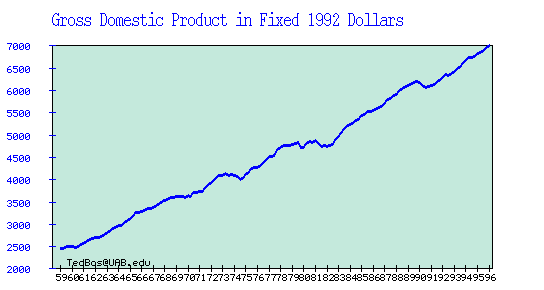FIGURE 2. Per Capita GDP: International Comparisons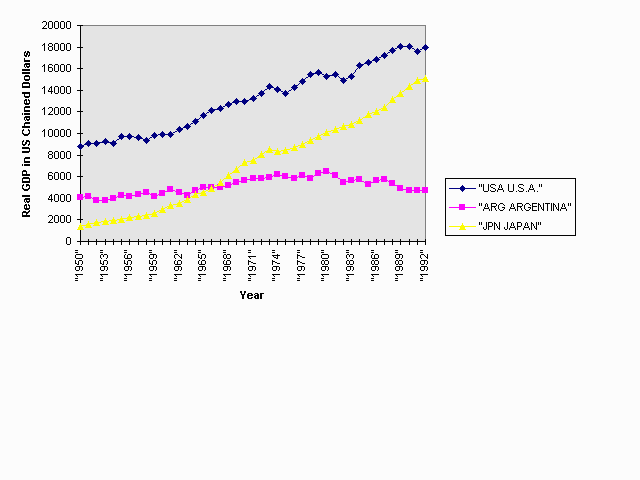Figure 3. Per Capita GDP: International Comparison 2FIGURE 4FIGURE 4'Figure 5. GNP Growth in Germany and Japan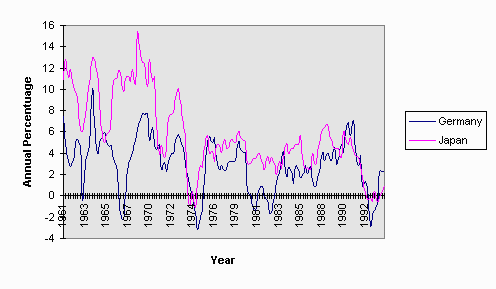Figure 6
Nominal and Real GDP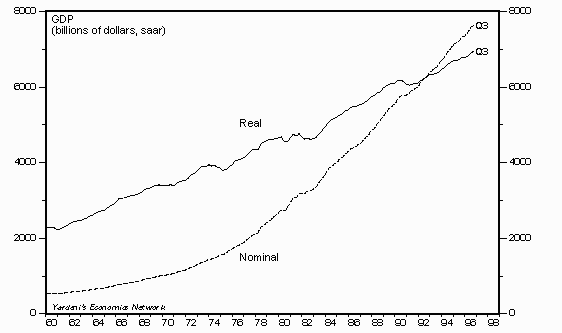Figure 7
Nominal and Real Growth Rate of GDPFigure 8. CPI Level
and its Percentage Annual Rate of Change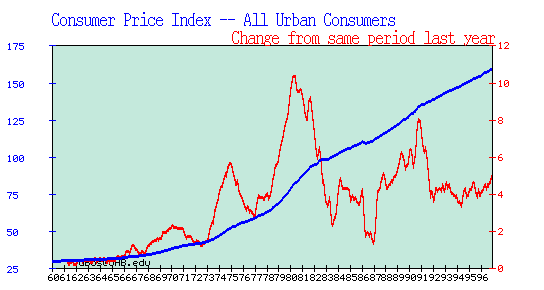Figure 9. GNP Deflator Index
and its Percentage Annual Rate of Change